# How large of an army could you make with the first ‘human-level’ AGIs?

There’s a popular prosaic alignment strategy that goes something like this:

1. Build human-level AGI via imitation, feedback, adversarial training, interpretability tools, etc.

2. Copy it loads of times.

3. Use your AGI army to solve alignment, take over the world, or do whatever needs to be done.

I’ve had a couple of conversations recently about whether such a collective would competent enough to perform a pivotal act.

An important crux seems to be: “how many copies could we run and how fast would we be able to run them?” If we had one billion human equivalents running at one million times the speed of a normal human, I would feel pretty good about our chances. But those are made-up numbers. Here are some Firmi estimates I came up with:

• The number of years of work the AGI copies would do per day if we ran them using the training compute alone:

* My 80% CI spans 3 − 6 orders of magnitude for each year (wider intervals for later years).

For reference, only 547 years of AI capabilities research and around .2 years of safety research are done per day.

• The cost of AGI labor:

• Speed of an individual AGI copy: probably at least 100x faster than a biological human, although this number could easily be much larger. This quantity can be used to calculate the number of copies you could run:

In the year 2050 (which is Ajeya’s median timelines estimate), you could run somewhere around 1,000,000 copies, each at 100x the speed of a human, using the training compute alone. Combining this tremendous magnitude of labor with other advantages AI systems will probably have, I think that aligned human-level AGI will probably be ‘enough.’

If you want to adjust the assumptions/​intermediate estimates, you can fiddle with this spreadsheet. The cruxiest number is probably FLOPs required to run a TAI model for one subjective second, which I’m taking to be 10^17 (Holden Karnofsky uses 10^14 for his estimate).

# Why all of this might be wrong

In addition to the uncertainties I’ve flagged in sections below, this entire framework might be wrong. Amplification might prove to be the cheapest way to scale capabilities, which means the first human-level AGI might be really big and inefficient.

According to the thousand brains theory, human intelligence emerges from an ensemble of cortical columns that are essentially copies of each other. Perhaps the first human-level AGI will be a bureaucracy of tiny models that have been trained to delegate (Daniel Kokotajlo has described a similar idea).

DeepMind’s PonderNet was trained to delegate to itself and therefore can be amplified by simply running it for more time. Also, deep learning successes like Alpha Fold, Alpha-code, and DALL-E 2 rely on hard-coded iteration that can be viewed as amplifying a weak learned model.

Applying massive amplification could be compute-intensive. So, we might develop a highly competent AGI that we can experiment with before it is economically viable to deploy.

# How many years of work would the TAI copies be able to do per day (using the compute from training)?

I’m going to pull a lot of the numbers from Ajeya’s timelines report, so I’ll use the term ‘TAI’ (transformative AI) instead of ‘AGI’ from here on out. I think her definition of TAI is close enough to what I mean by ‘human-level AGI’ for the purposes of these calculations.

I’m assuming that the training compute can easily be repurposed for running copies of the TAI, which I think is reasonable since training basically involves running the model a bunch (doing a lot of forward passes).

I’ll need three numbers:

1. X: The amount of compute required for training.

2. Y: The amount of compute required for a copy to do one year of work.

3. Z: The amount of time training takes assuming full usage of the compute (in days).

The number of years of work done per day by all copies combined is then ~ X/​(YZ)

## The amount of compute used for training

If we assume that TAI will be built as soon as it is affordable, we just need to know how much compute costs and what actors are willing to spend. Those quantities are shown below and are justified here: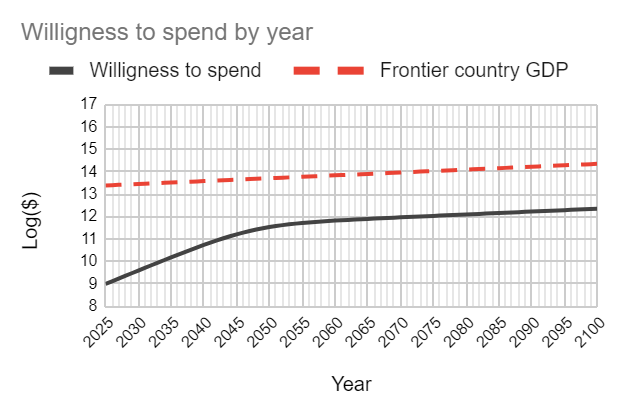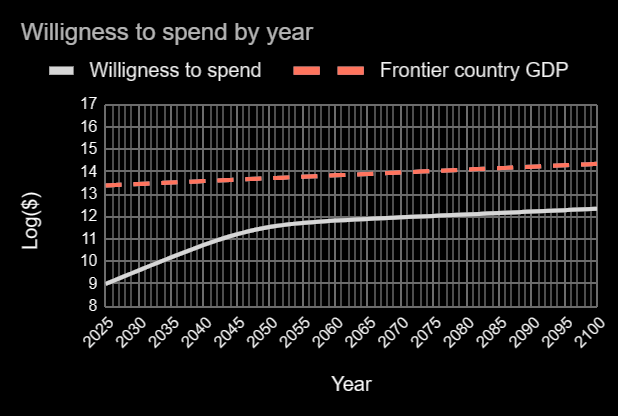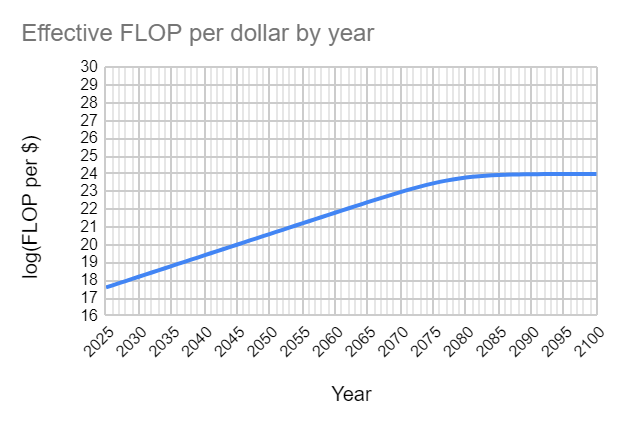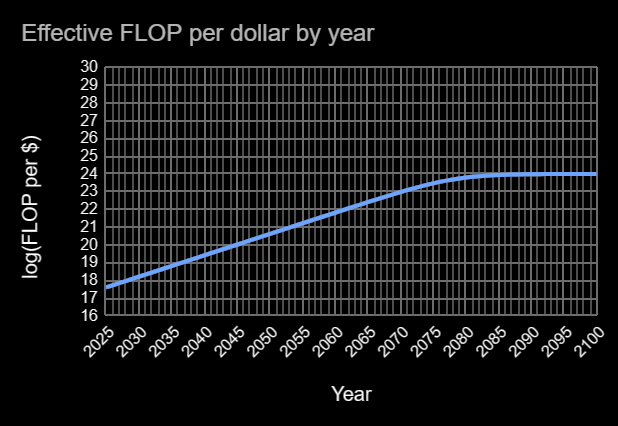Multiplying them yields FLOP used for training: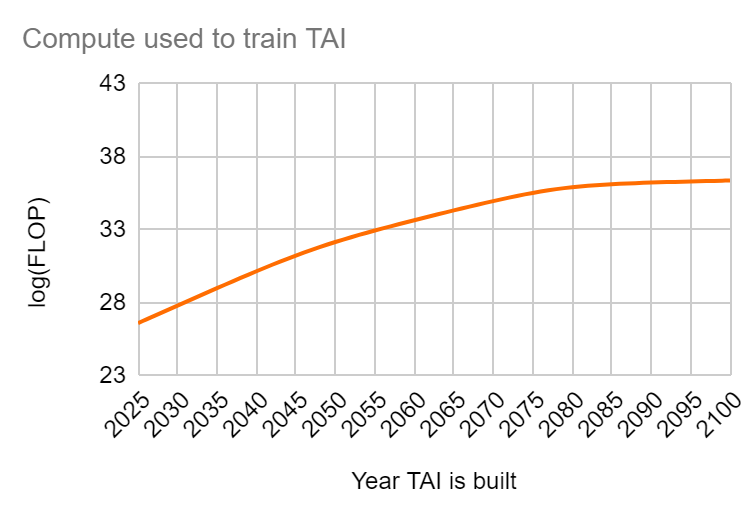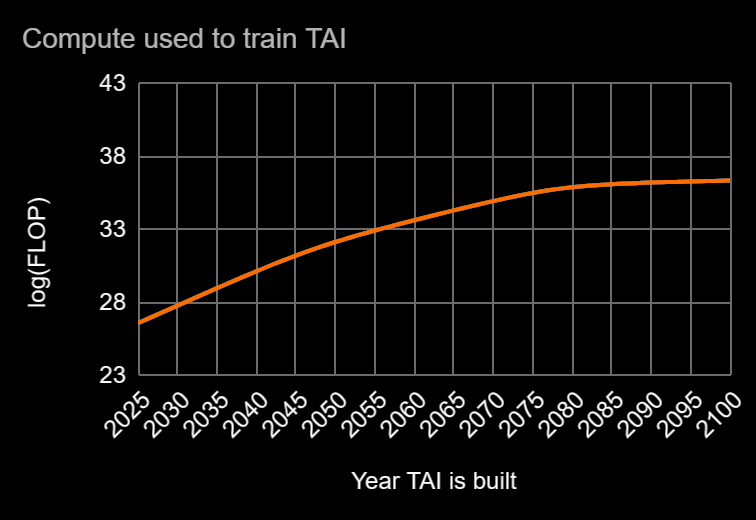## The amount of compute needed for one copy to do one year of work

Here’s Ajeya’s distribution for the FLOP done by the first TAI model per subjective second. The mean is 10^17 which translates to 3.17e9 FLOP per subjective year.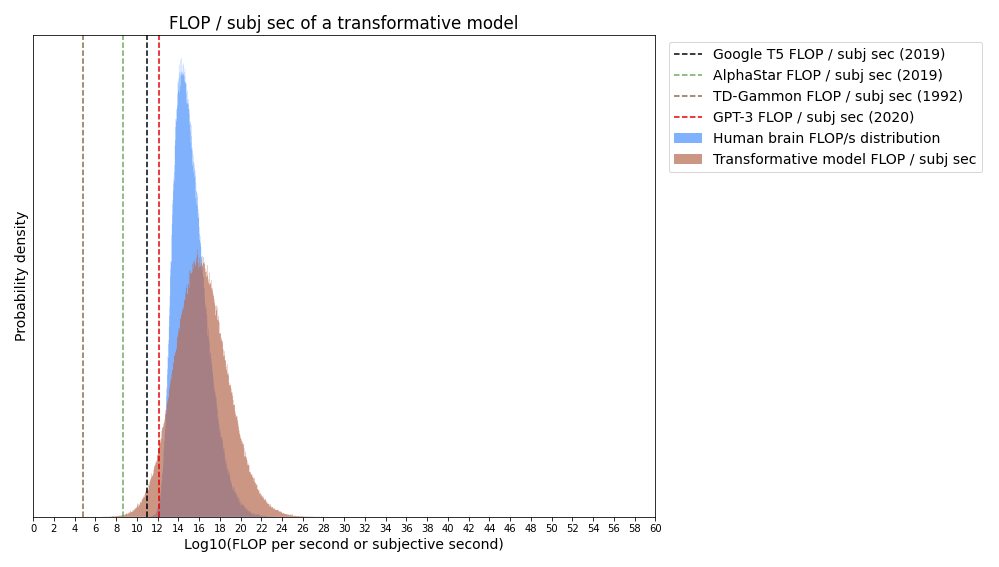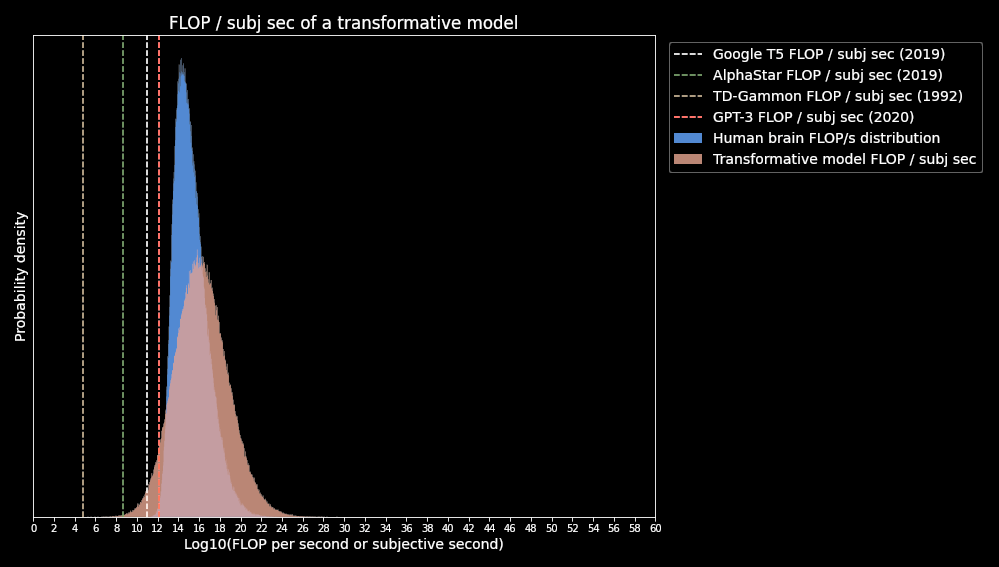## The amount of time that training takes assuming full usage of the compute (days).

My median estimate is ~ 60 days. The precision of this number is not very consequential since my uncertainty over the other quantities spans over several orders of magnitude.

Putting everything together yields the following curve for subjective years of work done per day: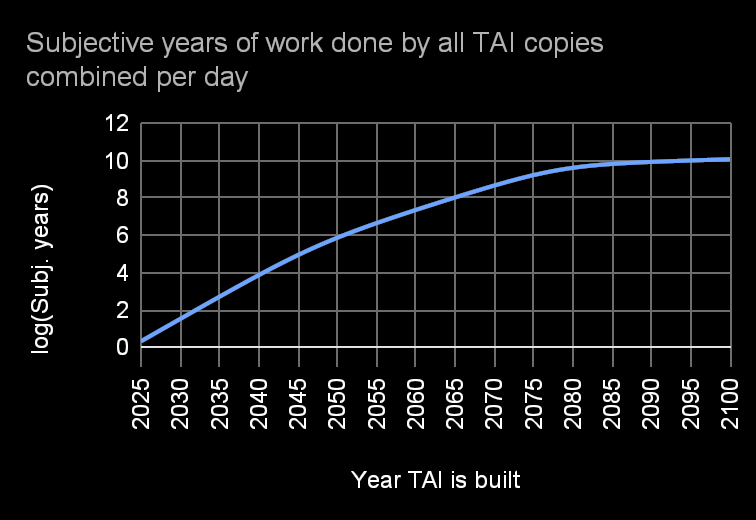This is just using the compute from training. If I assume that actors will be willing to spend 1% of the U.S. GDP running AGI copies, the curve looks like this: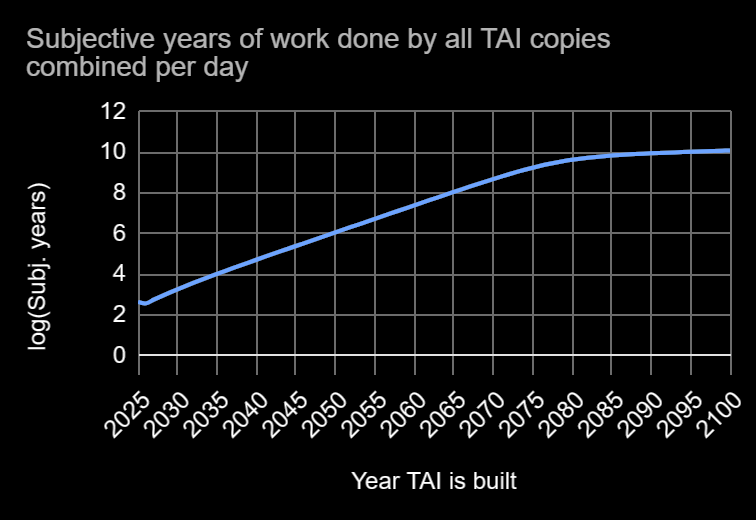To approximate my uncertainty for these estimates, I’ll first estimate the standard deviations of my credence distributions over the input quantities:

log(Training FLOP): 1 OOM

log(FLOP /​ subj second): 2 OOM

log(Training time): .5 OOM

Which implies:

2.98 orders of magnitude is a wide margin, but if we get TAI after 2040, the army of AGI copies should still be a force to be reckoned with.

# How much would it cost to run the TAIs?

I expect energy to be the primary variable cost of running the deployed models. Here’s a plot of GPU energy efficiency over time: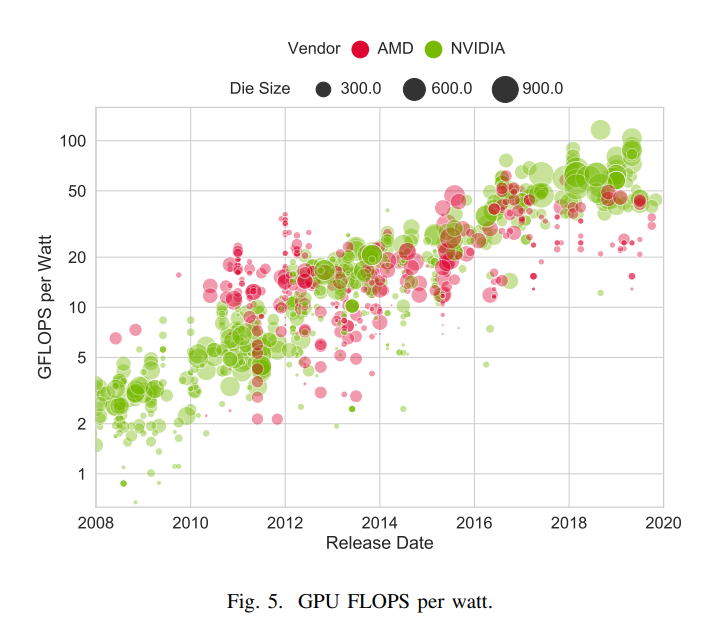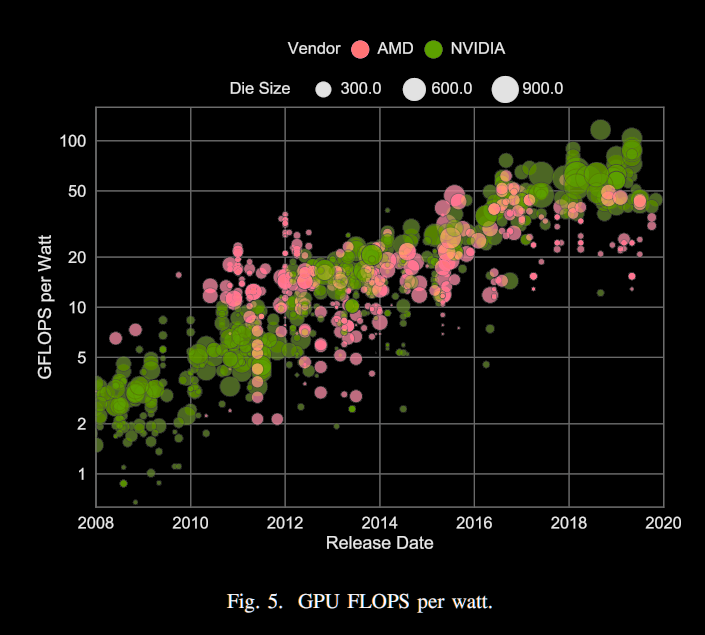Energy efficiency has doubled every ~2.12 years. I’ll project this trend into the future and upper bound it according to Landauer’s limit, which implies that the minimum energy required to flip one bit at room temperature is around 2.8E-21 J. Since a standard FLOP requires 32 bit flips, this translates to an upper bound of 1.12E+19 FLOP per Watt.

TPU v4 chips currently do 1.6E12 FLOPS per watt, so I expect FLOP/​watt to be around 3.2E12 in 2025.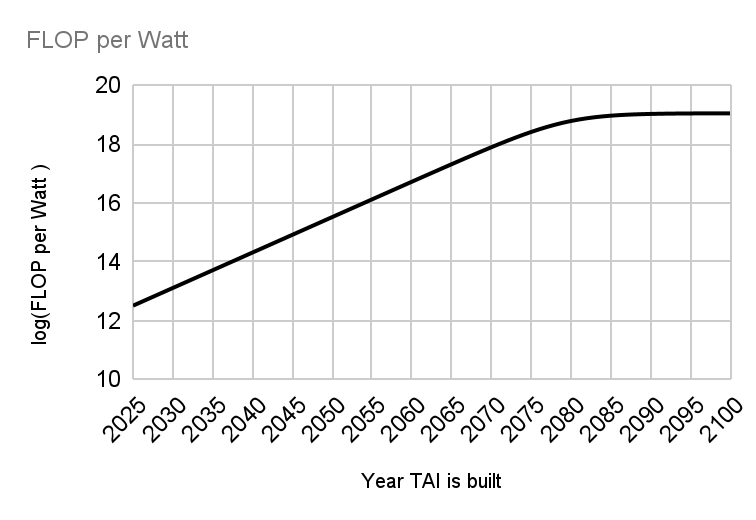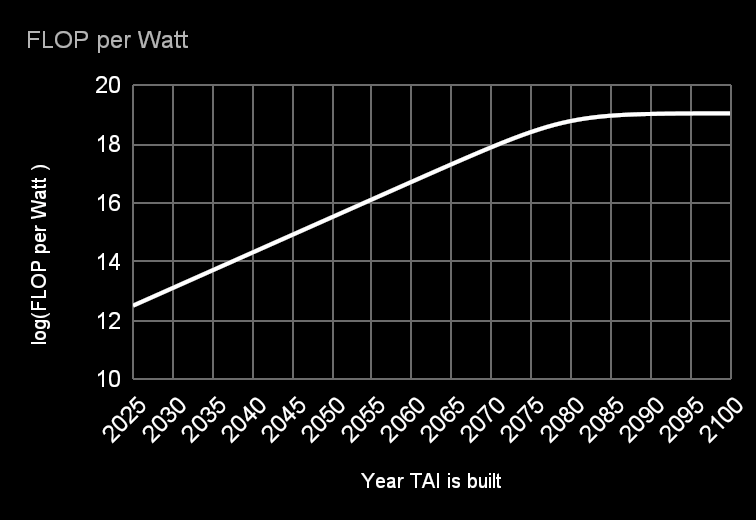Data center energy costs are currently around $0.05 per KW hour. I’ll be conservative and assume this cost to be fixed (even though I expect it to decrease). According to these estimates, the cost of running the deployed models should be quite low compared to hiring humans. If TAI was built today, it should be possible to run it for around$3.13 per subjective hour.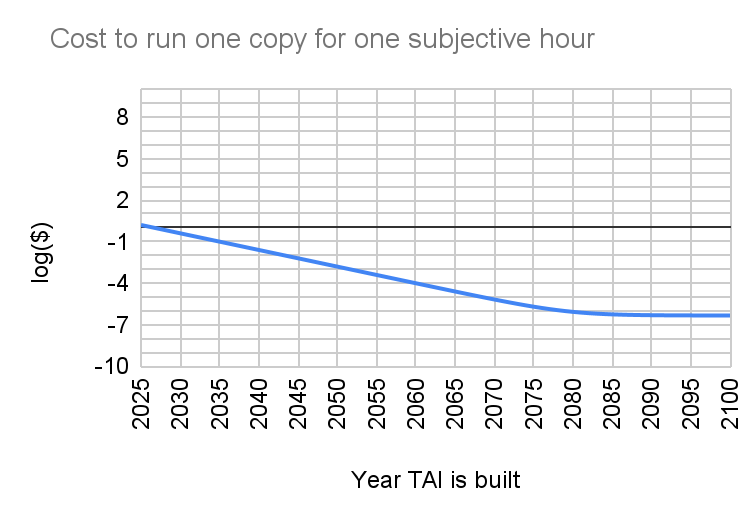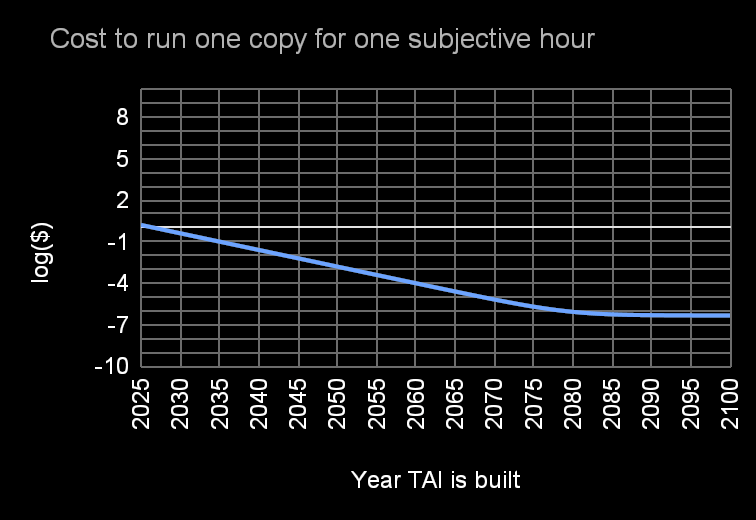# How quickly will these models run?

Serial computation is different from parallel computation. If you tell one billion humans to solve an IMO problem in one second, they would not have a chance. Heck, they probably wouldn’t even be able to add two three-digit numbers together. If you gave a single human one billion seconds (31 years) to solve that problem, it should be a piece of cake.

Research often builds off of past research, which means it is the kind of problem that benefits from serial computation. If we want human-level AGIs to build aligned superintelligence for us, it might be important for them to think much faster than we can.

I’m going to estimate inference speed via two methods:

1. Estimating how quickly a human brain emulation would run.

2. Estimating the speed of current ML models and extrapolating to TAI via scaling laws.

## The brain method

I’ll need two numbers:

1. X: The speed of computer chips measured in serial FLOPS. Note that if 1000 FLOPs happen simultaneously, that only counts as one ‘serial FLOP.’

2. Y: The number of serial FLOPs required to run a brain for one subjective second.

The speed multiplier is then X/​Y.

### Computer chip speed

A CPU or GPU core carries out one instruction at a time in what is called a cycle. The below plot shows CPU clock frequency, which is the number of cycles per second: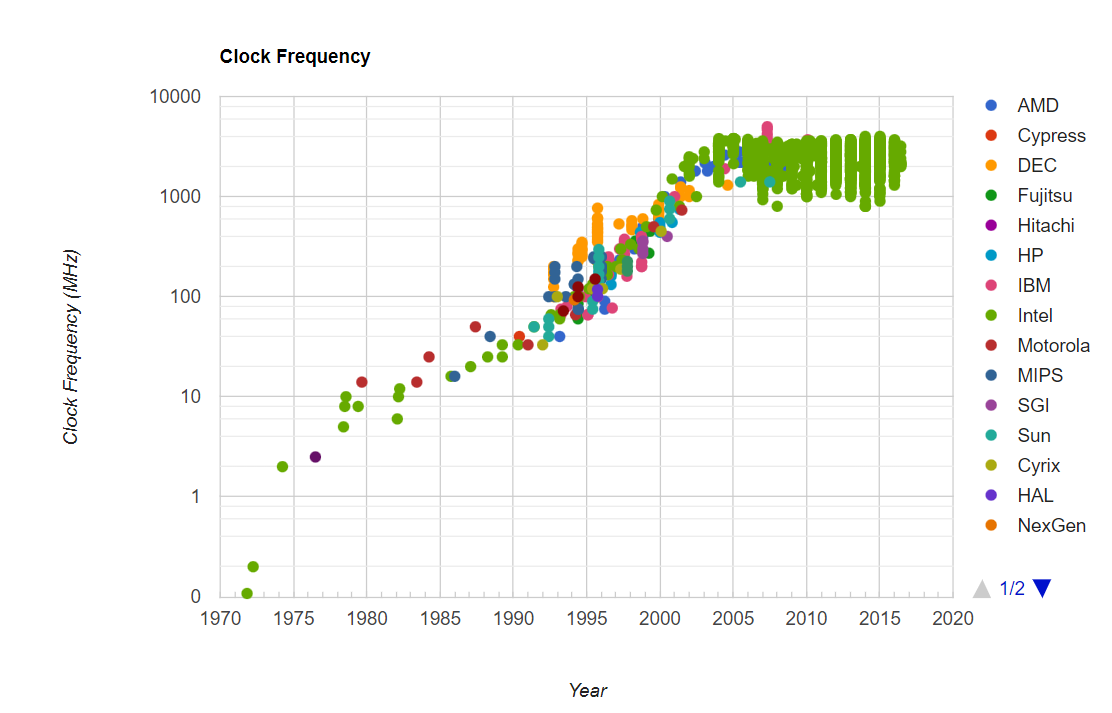Clock frequency leveled off in around 2005 at around 5.5GHz – apparently as a result of engineering difficulties that emerge at atomic scales (transistor gates are close to 1 atom thick). Since a fused multiply-add instruction involves 2 FLOPs, 5.5GHz corresponds to 1.2E10 serial FLOPs per second.

GPU clock speed continues to increase – although growth has been slowing down and it appears to gradually be approaching 5.5GHz.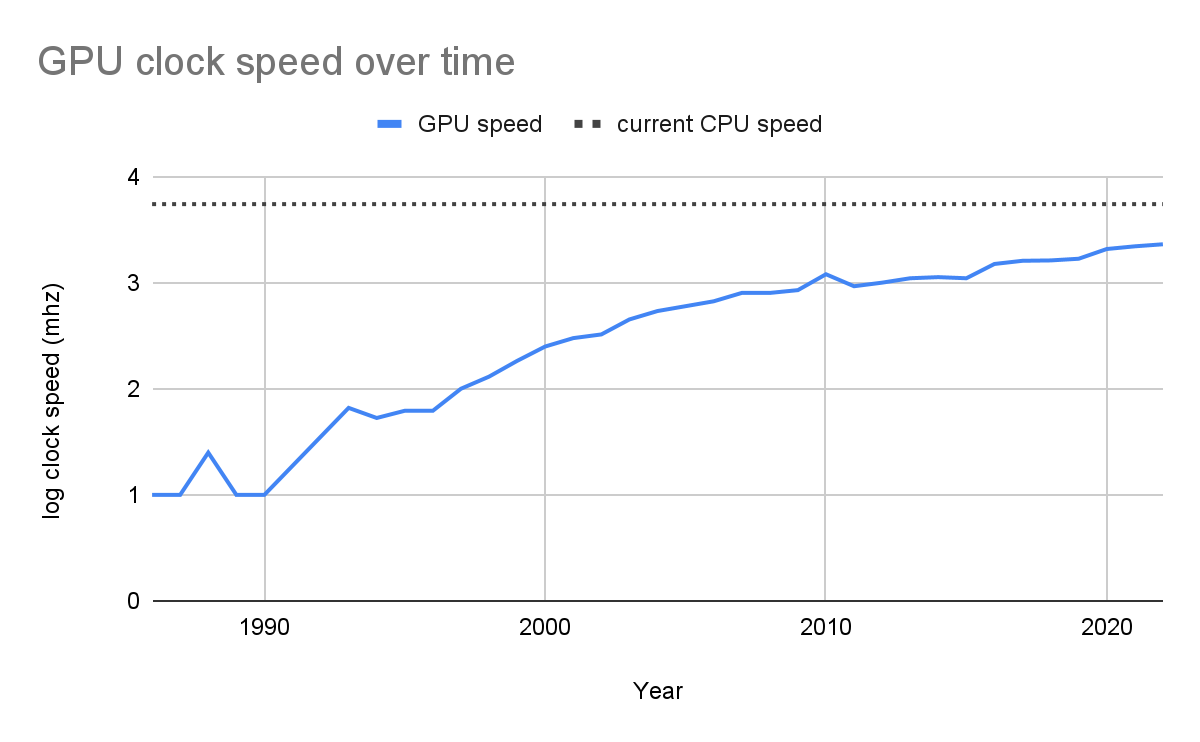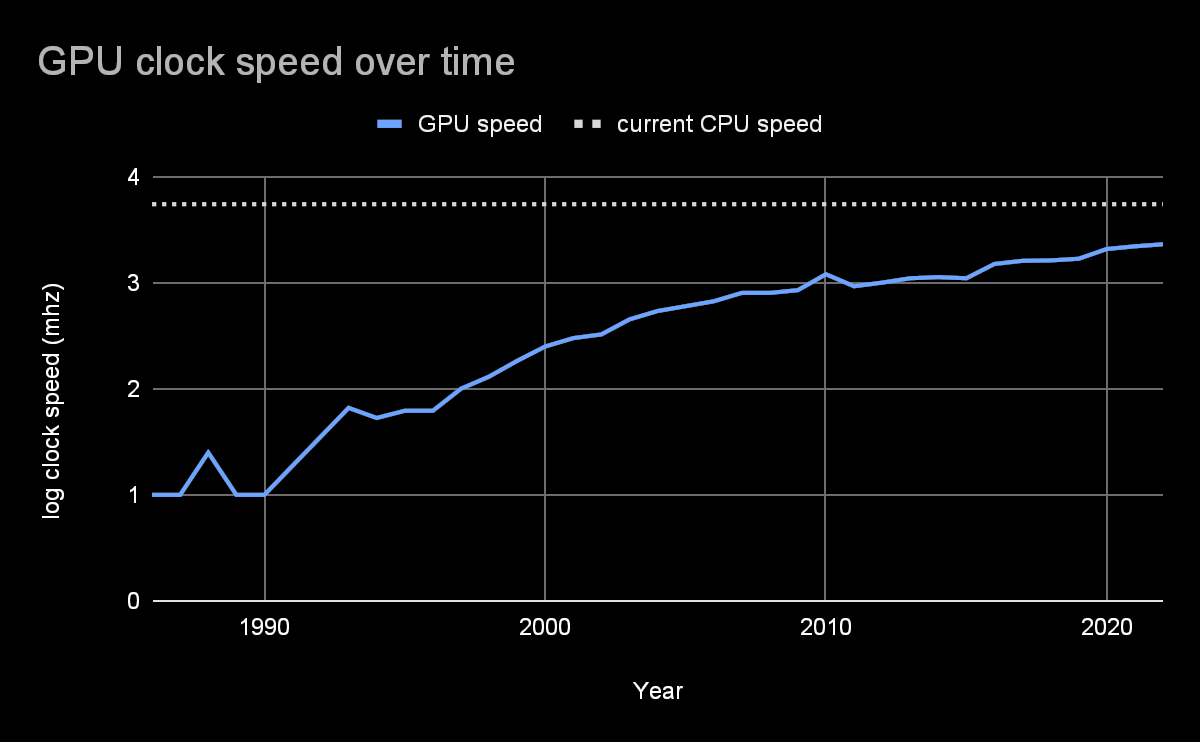I’ll be conservative and assume that 1.2E10 FLOPs per second is the maximum serial speed we are going to achieve. Note that total FLOPs per second and FLOPs per dollar have increased as a result of greater parallelization and economies of scale, so this serial speed limit would probably not be a barrier to building TAI. It just affects how quickly deployed models will be able to run.

### Serial FLOPs needed to run a brain for one second.

People often cite the fact that neurons have a max firing rate of 100hz to support the claim that the brain does not do a lot of serial computation. This justification is not quite right since the computational path could still be quite long if it goes through neurons that have not fired recently.

The more relevant quantity is transmission speed. It takes 0.5ms − 1 ms for a chemical signal to diffuse across a synapse, which means that the maximum number of sequential neuron firings that can happen in the brain per second is 2000. An OpenPhil report estimates that each spike through the synapse corresponds to approximately 1-100 FLOP of computation. This implies that only ~ 2,000-200,000 serial FLOPs per second are performed by the human brain.

By this estimate, a brain emulation could be run at ~ 60,000 − 6,000,000x the speed of a physical brain. Of course, this number should be lower after you account for network/​memory latencies and the fact that not all computation that can be done in parallel generally is done in parallel.

## Extrapolating from current models

A surprisingly small number of serial FLOPs are needed to do a forward pass of a transformer. Here is an approximate formula for the number of serial FLOPs required to do a forward pass with a decoder-only model:

is the number of decoder blocks, is the context length, is the embedding dimension, is the width of the fully connected net, is the number of rows in the value matrix (for attention), is the number of attention heads, and is the output dimension.

According to this formula, only 19,000 serial FLOPs are required to do a forward pass of Chinchilla. That means it should take 19,0001.2E10 = 2E-6 seconds = .002 ms to do one forward pass. In the real world, GPUs don’t do every operation in parallel and there are other latencies involved. Jacob Steinhardt takes these considerations into account in his post how fast can we perform a forward pass and estimates that Chinchilla completes a forward pass in 0.7 ms. Humans type 40 words per minute on average, so Chinchilla is 1050x faster than a human at writing.

Note that the serial FLOP count increases with the log of model width parameters. Since scaling laws depend on parameter count but very weakly on shape, this means that models can be scaled up significantly and take a similar amount of time to run!!

Ajeya’s central estimate for the number of parameters the first TAI model will have is 3E14 and chinchilla has 7E10. Scaling up 10,000x would produce a model with around 3E14 parameters, and that would only add around 4250 to the minimum serial FLOP count.

So theoretically, transformer models could continue to be extremely fast, even as we scale them up—although I don’t know enough about the engineering side of things to say whether there will be other bottlenecks.

My tentative estimate is that the first TAI model will be at least 100x faster than a human, although this number could easily be much larger since there is a lot of room for parallelization to improve.

1. ^

This is assuming 200k AI researchers/​engineers and ~100 AI safety researchers.

2. ^

Subjective years of work done per day = (training FLOP)/​[(training days)*60*60*24*365*(FLOP needed for one subjective second)]

3. ^

I was not able to find the value of in the original paper, so I assumed it to be the same as .

4. ^

• If an army of human level AGIs could work together to solve problems we currently can’t superhumanly fast, then they combined would effectively be an AGI, and we would have to make sure they were aligned with us first.

• I know that this is a common argument against amplification, but I’ve never found it super compelling.

People often point to evil corporations to show that unaligned behavior can emerge from aligned humans, but I don’t think this analogy is very strong. Humans in fact do not share the same goals and are generally competing with each other over resources and power, which seems like the main source of inadequate equilibria to me.

If everyone in the world was a copy of Eliezer, I don’t think we would have a coordination problem around building AGI. They would probably have an Eliezer government that is constantly looking out for emergent misalignment and suggesting organizational changes to squash it. Since everyone in this world is optimizing for making AGI go well and not for profit or status among their Eliezer peers, all you have to do is tell them what the problem is and what they need to do to fix it. You don’t have to threaten them with jail time or worry that they will exploit loopholes in Eliezer law.

I think it is quite likely that I am missing something here and it would be great if you could flush this argument out a little more or direct me towards a post that does.

• typo: Ajaya should be Ajeya

• Thanks!

• Humans are trained on a tiny unique subset of available training data. I would expect multiple instances of a set of AI software trained on close to the same set of data to think very similar to each-other, and not provide more creative capability than a single AI with more bandwidth.

• That’s a good point. I guess I don’t expect this to be a big problem because:
1. I think 1,000,000 copies of myself could still get a heck of a lot done.
2. The first human-level AGI might be way more creative than your average human. It would probably be trained on data from billions of humans, so all of those different ways of thinking could be latent in the model.
2. The copies can potentially diverge. I’m expecting the first transformative model to be stateful and be able to meta-learn. This could be as simple as giving a transformer read and write access to an external memory and training it over longer time horizons. The copies could meta-learn on different data and different sub-problems and bring different perspectives to the table.